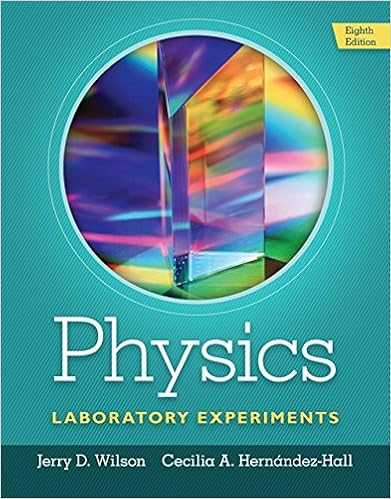# Lab 2.docx - 1.2 Pre-lab questions 1 What is the basic...

• Lab Report
• 6
• 50% (2) 1 out of 2 people found this document helpful

This preview shows page 1 - 3 out of 6 pages.

##### We have textbook solutions for you!
The document you are viewing contains questions related to this textbook.The document you are viewing contains questions related to this textbook.
Chapter 6 / Exercise 8
Physics Laboratory Experiments
WilsonExpert Verified
1.2 Pre-lab questions1) What is the basic difference between scalars and vectors?
2) Is temperature is a vector quantity? What do plus and minus temperature signify? Explain.
3) A vector A has a magnitude of 60 m and directed as shown.Find the xand ycomponents of this vector.
4) What are, briefly, the steps to find the resultant of vectors?
5.The resultant vector is the addition of the the x-components (Ax) and the y-components (Ay)
5) What is the difference between a resultant & equilibrant vector & what do they physically mean?
##### We have textbook solutions for you!
The document you are viewing contains questions related to this textbook.The document you are viewing contains questions related to this textbook.
Chapter 6 / Exercise 8
Physics Laboratory Experiments
WilsonExpert Verified
Physically, the equilibrant force will match the resultant in the opposite direction in order for the particle to reach equilibrium.2. The instruction pages2.1 The Purpose / or Objectives of the experimentThe purpose of this experiment is to understand how to (1) find resultant vectors in with anumber of forces acting on the force table, (2) calculate the resultant of the same force by drawing a vector diagram to scale to find its magnitude and direction, and (3) find the resultant using the analytical method, including using law of cosine for magnitude and law of sine for its direction.
•••